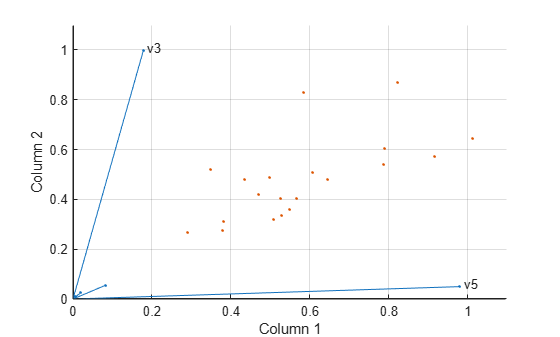Documentation

## Perform Nonnegative Matrix Factorization

This example shows how to perform nonnegative matrix factorization.

```load moore X = moore(:,1:5); rng('default'); % For reproducibility```

Compute a rank-two approximation of `X` using a multiplicative update algorithm that begins from five random initial values for `W` and `H`.

```opt = statset('MaxIter',10,'Display','final'); [W0,H0] = nnmf(X,2,'replicates',5,'options',opt,'algorithm','mult');```
``` rep iteration rms resid |delta x| 1 10 358.296 0.00190554 2 10 78.3556 0.000351747 3 10 230.962 0.0172839 4 10 326.347 0.00739552 5 10 361.547 0.00705539 Final root mean square residual = 78.3556 ```

The `'mult'` algorithm is sensitive to initial values, which makes it a good choice when using `'replicates'` to find `W` and `H` from multiple random starting values.

Now perform the factorization using alternating least-squares algorithm, which converges faster and more consistently. Run 100 times more iterations, beginning from the initial `W0` and `H0` identified above.

```opt = statset('Maxiter',1000,'Display','final'); [W,H] = nnmf(X,2,'w0',W0,'h0',H0,'options',opt,'algorithm','als');```
``` rep iteration rms resid |delta x| 1 2 77.5315 0.000830334 Final root mean square residual = 77.5315 ```

The two columns of `W` are the transformed predictors. The two rows of `H` give the relative contributions of each of the five predictors in `X` to the predictors in `W`. Display H.

`H`
```H = 2×5 0.0835 0.0190 0.1782 0.0072 0.9802 0.0559 0.0250 0.9969 0.0085 0.0497 ```

The fifth predictor in `X` (weight 0.9802) strongly influences the first predictor in `W`. The third predictor in `X` (weight 0.9969) strongly influences the second predictor in `W`.

Visualize the relative contributions of the predictors in `X` with `biplot`, showing the data and original variables in the column space of `W`.

```biplot(H','scores',W,'varlabels',{'','','v3','','v5'}); axis([0 1.1 0 1.1]) xlabel('Column 1') ylabel('Column 2')```